Software DevelopmentLearn How To Run Machine Learning Algorithms Using Spark

# Learn How To Run Machine Learning Algorithms Using Spark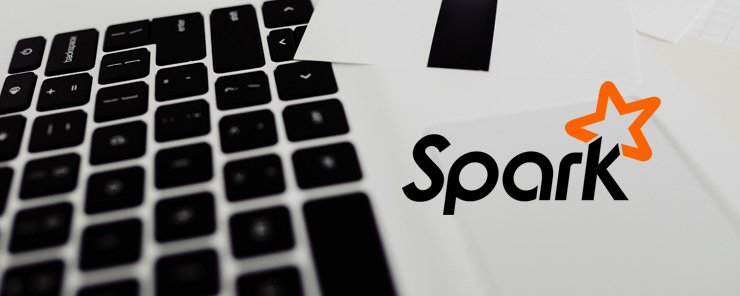This tutorial builds on previous tutorials that demonstrated how to set up an Amazon cluster running spark and how to install Spark on a local machine. If you have not set up Spark please refer to learn how to set up Spark tutorial.

In machine learning there are three categories of problems that can be solved. These are classification, clustering and recommender systems (collaborative filtering).

Machine learning algorithms can also be classified as supervised or unsupervised. Supervised algorithms are given data that has been categorized from which they learn to use new data points to predict their category. Data that has already been categorized is referred to as the training data set. An iterative process of improving the accuracy of a algorithm to an acceptable level is used to develop a prediction model. With supervised algorithms you can use binary/multiclass classification or linear regression. Binary classification is used when you have two distinct categories to predict. For example you can use it to predict if a transaction is fraudulent or not. Algorithms available in Spark for doing binary classification are logistic regression, decision trees and random forests. Linear support vector machines (SVM). When you have more than two distinct categories you use multiclass classification. Available algorithms for multiclass classification are logistic regression, decision trees, random forests and naive bayes. When you would like to predict a continuous instead of binary variable you use linear regression. Algorithms available are linear, ridge, lasso regression, decision trees and random forests.

Unsupervised algorithms are not given any data with known categories instead they infer the structure of the data and make predictions based on that. Problems solved with these algorithms are clustering, reducing data dimensionality and learning association rules. For doing clustering k-means, power iteration clustering (PIC) and latent Dirichlet allocation (LDA) algorithms are available.

Data dimensionality reduction is used when you would like to reduce a very large number of variables but still retain signal contained in the data. Available algorithms are principal component analysis (PCA) and singular value decomposition (SVD). For dealing with recommender systems problems Spark avails alternate least squares (ALS) algorithm. Each of the algorithms mentioned here has a set of parameters that can be changed in the iterative model building process. For an exhaustive discussion of these parameters refer to Spark documentation available online.

Machine learning algorithms in Spark are found in spark.mlib and spark.ml. Spark.mlib operates on resilient distributed datasets (RDD) while spark.ml operates on the newer DataFrame API. Interactive running of algorithms is possible using Python and Scala shells bundled with Spark.

In this tutorial we will demonstrate how to run a logistic regression model which is a supervised learning algorithm. We will also show how to use a k-means algorithm for data clustering. For the logistic regression model we will use titanic data set to demonstrate how to fit a model. The csv data file is available here http://biostat.mc.vanderbilt.edu/wiki/Main/DataSets. The data contains a binary variable that shows if a passenger survived or not. It also has several variable that can be used to predict the category of a passenger.

To access Scala shell use this command spark-shell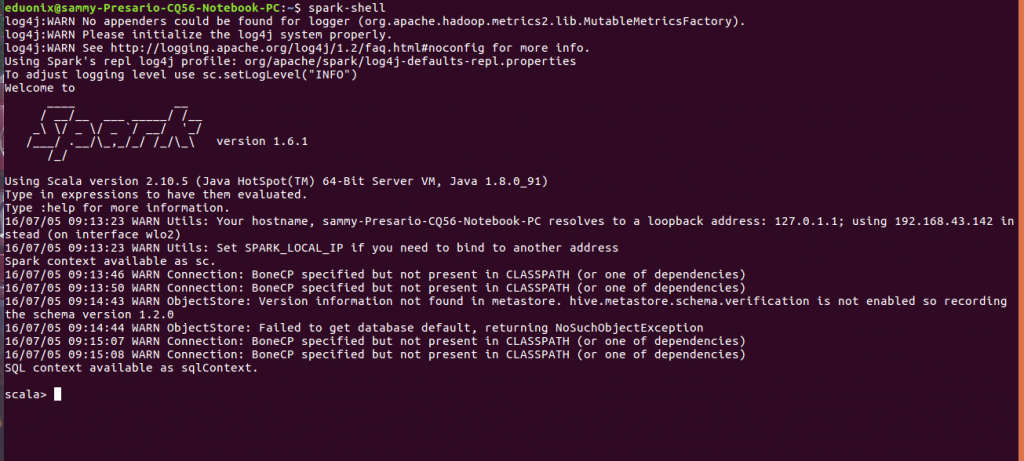Spark provides two APIs for working with data. You can use the RDD or the data frame API. When you are using the RDD API machine learning algorithms are available in the spark.mlib package. When you are using the data frame API machine learning algorithms are available in spark.ml package.
Read in the data into a Spark RDD using the command below. After loading data and creating an RDD you use spark.mlib package to create your models.

Use the command below to load the titanic3.csv file that was downloaded and saved in downloads directory.

`val titanicdata = sc.textFile("/home/eduonix/Downloads/titanic3.csv")`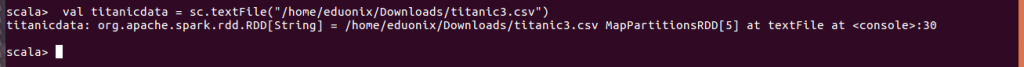Grab the first row to observe the column names using the command below.

`titanicdata.take(1).map(println)`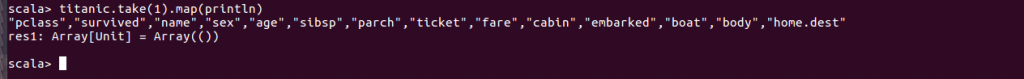By default Spark loads the data as a string RDD. Such a data set is not suitable for statistical analysis and modeling. We need to create a schema that schema that specifies the data type of each column. We also need to convert the columns from the string data type to their respective data types. Commonly used data types are String, Integer and Float. For each of the columns in the titanic RDD we need to specify its data type. A case class is used to define a schema using structure shown below.

```import sqlContext.implicits._
case class titanicdata(pclass: Integer, survived: Integer, name: String, sex: String, age:Float,	sibsp: Integer, 	parch: Integer, ticket: Float, fare: Float, cabin: String,	embarked: String, boat: String,	body: Float, homedest: String)```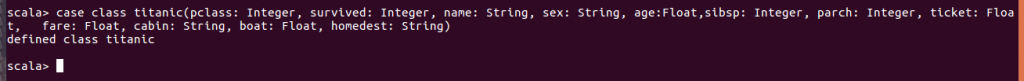Once the schema has been created we use it to convert our string RDD using the construct shown below. You specify the rdd to be operated on and how each of the columns is to be converted. The notation p(0) is used to reference the columns.

```val titanicDefined = titanicdata.map(_.split(",")).map(p => titanic(p(0),p(1).toInteger,p(2),p(3).toString,p(4).toFloat,p(5),p(6).toInteger,
p(7),p(8).toFloat,p(9),p(10),p(11).toString,p(12).toFloat,p(13).toString))```

Having created a RDD with the correct schema we convert it to a data frame using the manner shown below.

`val titanicDf = titanicDefined.toDF()`

When training a model you split available data into training and test sets. The training set is used to develop the model while the test set is used to evaluate accuracy of developed model. For example to split the data frame created above into 60% of the data for training and 40% for training the construct shown below is used.
First import required packages

```import org.apache.spark.mllib.classification.{LogisticRegressionWithLBFGS, LogisticRegressionModel}
import org.apache.spark.mllib.evaluation.MulticlassMetrics
import org.apache.spark.mllib.regression.LabeledPoint
import org.apache.spark.mllib.linalg.Vectors
import org.apache.spark.mllib.util.MLUtils```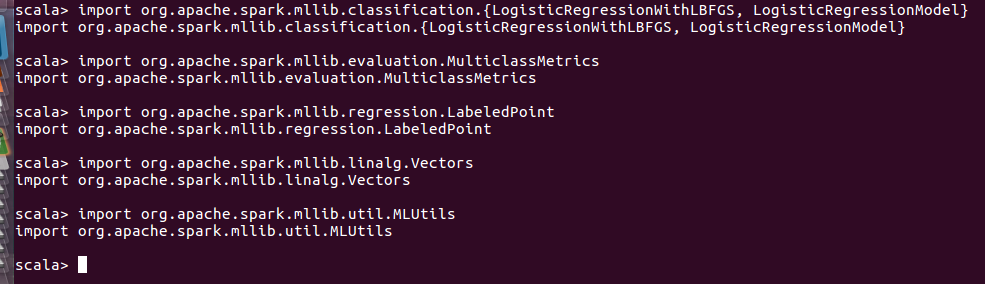Data splitting is done as shown below

```val splitdata = titanicDf.randomSplit(Array(0.6, 0.4), seed = 11L)
val training = splitdata(0).cache()
val test = splitdata(1)```

In logistic regression you need to specify a binary variable and a set of predictors. Creating the binary variable is referred as labeling.
A regression model is developed using syntax shown below

```val model = new LogisticRegressionWithLBFGS()
.setNumClasses(2)
.run(training)```

This tutorial explained the various types of machine learning algorithms that are available in Spark. The two packages, spark.mlib and spark.ml that contain algorithms were introduced and use of each was discussed. The algorithms have parameters that are used to tune them which could not be covered in this tutorial because of their expansiveness. Proper use of algorithms requires a good level of understanding statistics. Documentation available online discusses these issues therefore it is important to review it for a thorough presentation of all concepts.

### Is It Possible To Get A High-Paying Tech Job Straight Out Of High School?

21,501Fans
4,210Followers
104,000Subscribers

### Is It Possible To Get A High-Paying Tech Job Straight Out Of High School?

- Advertisement -